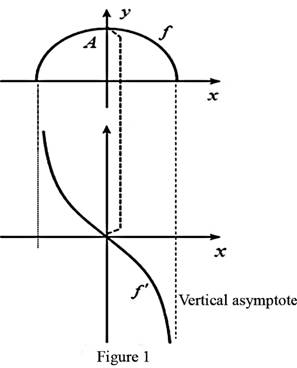# The graph of f ′ below the graph of f.### Single Variable Calculus: Concepts...

4th Edition
James Stewart
Publisher: Cengage Learning
ISBN: 9781337687805### Single Variable Calculus: Concepts...

4th Edition
James Stewart
Publisher: Cengage Learning
ISBN: 9781337687805

#### Solutions

Chapter 2.7, Problem 7E
To determine

## To sketch: The graph of f′ below the graph of f.

Expert Solution

### Explanation of Solution

From the given graph, it is observed that the graph of f contains the horizontal tangent at one point. Let the point be A.

Note that, the value of the derivative will be zero at the point where the function has the horizontal tangent.

Thus, the graph of f will be zero at the points A.

From the given graph, f has a vertical tangent line at the left end point. This implies that, the derivative function f has a vertical asymptote at that point. Thus, f is not differentiable at that point.

From the point A to left, the slope of the graph f is positive which implies that the derivative graph f must have a functional value in positive.

From the point A to right, the slope of the graph f is negative which implies that the derivative graph f must have a functional value in negative.

From the given graph, f has a vertical tangent line at the right end point. This implies that, the derivative function f has a vertical asymptote at that point. Thus, f is not differentiable at that point.

Graph:

Use the above information and obtain the graph of f(x) as shown below in Figure 1.Thus, f is the required graph.

### Have a homework question?

Subscribe to bartleby learn! Ask subject matter experts 30 homework questions each month. Plus, you’ll have access to millions of step-by-step textbook answers!